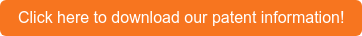# The Lumenarium: The official blog of Lumetrics, Inc.

Posted June 26, 2019 by Kristin

Abstract: A method of identifying the material and determining the physical thickness of each layer in a multilayer structure is disclosed.The method includes measuring the optical thickness of each of the layers of the multilayer object as a function of wavelength of a light source and calculating a normalized group index of refraction dispersion curve for each layer in the multilayer structure.  The measured normalized group index of refraction dispersion curves for each of the layers is then compared to a reference data base of known materials and the material of each layer is identified.  The physical thickness of each layer is then determined from the group index of refraction dispersion curve for the material in each layer and the measured optical thickness data.  A method for determining the group index of refraction dispersion curve of a known material is also disclosed.